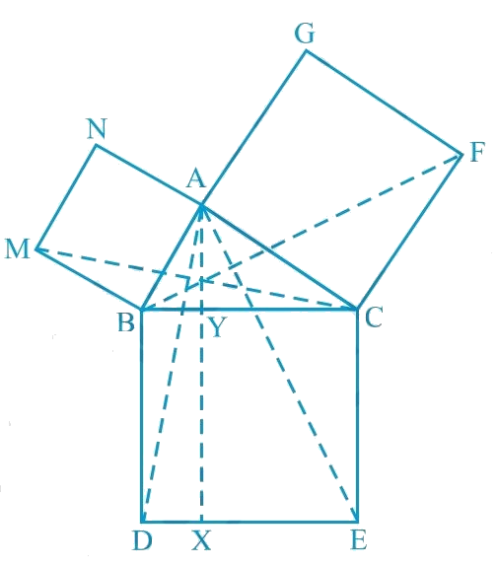# Ex.9.4 Q8 Areas of Parallelograms and Triangles Solution - NCERT Maths Class 9

Go back to  'Ex.9.4'

## Question

In the following figure, $$ABC$$ is a right triangle right angled at $$A$$. $$BCED$$, $$ACFG$$ and $$ABMN$$ are squares on the sides $$BC$$, $$CA$$ and $$AB$$ respectively. Line segment $$AX ⊥ DE$$ meets $$BC$$ at $$Y$$.

##Show that:

(i) \begin{align}\Delta MBC \cong \Delta ABD \end{align}

(ii) \begin{align}\operatorname{ar}(BYXD)=2 \operatorname{ar}(MBC)\end{align}

(iii) \begin{align}\operatorname{ar}(BYXD)=2 \operatorname{ar}(ABMN)\end{align}

(iv) \begin{align}\Delta FCB \cong \Delta ACE \end{align}

(v) \begin{align}\operatorname{ar}(CYXE)=2 \operatorname{ar}(FCB)\end{align}

(vi) \begin{align}\operatorname{ar}(CYXE )= \operatorname{ar}(ACFG)\end{align}

(vii) \begin{align} \begin{bmatrix} \operatorname{ar}(BCED) \\ \begin{Bmatrix}= {\rm{ar}} (ABMN)\\ + \operatorname{ar}(ACFG)\end{Bmatrix} \end{bmatrix} \end{align}

Note: Result (vii) is the famous Theorem of Pythagoras. You shall learn a simpler proof of this theorem in class X.

Video Solution
Areas Of Parallelograms And Triangles
Ex 9.4 | Question 8

## Text Solution

#### Steps:

(i) We know that each angle in a square measures $$90^\circ$$.

Hence,

$\angle ABM =\angle DBC=90^{\circ}$

\begin{align} &\therefore \angle ABM+\angle ABC \\& \qquad=\angle DBC+\angle ABC \\& \therefore \angle MBC=\angle ABD \end{align}

In $$\Delta MBC$$ and $$\Delta ABD ,$$

$$\angle MBC$$ $$=$$ $$\angle ABD$$ (Proved above)

$$MB = AB$$ (Sides of square $$ABMN$$)

$$BC = BD$$ (Sides of square $$BCED$$)

\begin{align}\therefore \Delta MBC & \cong \Delta ABD \\ &\begin{pmatrix} \text { SAS } \\ \text{congruence} \\ \text{ rule } \end{pmatrix}\end{align}

(ii) We have

\begin{align} &\Delta MBC \cong \Delta ABD \\ \therefore \, & \operatorname{ar}(\Delta MBC) \\ & = \text{ar}(\Delta ABD) \!\! \ldots(1)\end{align}

It is given that $$AX ⊥ DE$$ and $$BD ⊥ DE$$ (Adjacent sides of square $$BDEC$$)

$$\therefore BD\; \| \; \mathrm{AX}$$ (Two lines perpendicular to the same line are parallel to each other.)

$$\Delta ABD$$ and parallelogram $$BYXD$$ are on the same base $$BD$$ and between the same parallels $$BD$$ and $$AX$$.

\begin{align} & \text {Area}(\Delta BYXD) \\ &=2 \text { Area }(\Delta MBC) \\ & \quad \begin{bmatrix} \text { Using} \\ \text{ equation }(1) \end{bmatrix} \ldots(2) \end{align}

(iii)  $$\Delta MBC$$ and parallelogram $$ABMN$$ are lying on the same base $$MB$$ and between same parallels $$MB$$ and $$NC$$.

\begin{align} &2 \operatorname{ar}(\Delta MBC) \\ & =\operatorname{ar}(ABMN) \\ \\ & \text { ar }(\Delta BYXD) \\ & =\operatorname{ar}(ABMN) \\ & \quad \begin{bmatrix} \text { Using } \\ \text{equation }(2) \end{bmatrix} \ldots(3)\end{align}

(iv) We know that each angle of a square is $$90^\circ$$.

\begin{align}&\therefore \angle FCA =\angle BCE =90^{\circ} \\& \therefore \angle FCA +\angle ACB \\ & \quad =\!\! \begin{pmatrix} \angle BCE \\ +\angle ACB \end{pmatrix} \end{align}

Adding $$\angle$$$$ACB$$ on both the sides

$$\therefore \angle FCB=\angle ACE$$

In $$\Delta FCB$$ and $$\Delta ACE$$,

\begin{align} \angle FCB &= \angle AC \\ & \quad \begin{pmatrix} \text{Sides of } \\ \text{square} \\ ACFG \end{pmatrix} \end{align}

$$CB = CE$$ (Sides of square $$BCED$$)

\begin{align} \Delta FCB & \cong \triangle ACE \\ & \quad \begin{pmatrix} \text { SAS } \\ \text{congruence} \\ \text{ rule } \end{pmatrix} \end{align}

SAS congruence rule- if two sides and the included angle of one triangle are equal to the two sides and the included angle of the other triangle then the triangles are congruent.

(v) It is given that $$AX ⊥ DE$$ and $$CE ⊥ DE$$ (Adjacent sides of square $$BDEC$$)

Hence, $$CE\;|| \;AX$$ (Two lines perpendicular to the same line are parallel to each other)

Consider $$\Delta ACE$$ and parallelogram $$CYXE$$

$$\Delta ACE$$ and parallelogram $$CYXE$$ are on the same base $$CE$$ and between the same parallels $$CE$$ and $$AX$$.

\begin{align} \therefore \, & \text{ ar }(\Delta CYXE) \\ & = 2 \text { ar }(\Delta ACE) \ldots(4) \end{align}

$$\therefore \Delta FCB \cong \triangle ACE$$

$$\operatorname{ar}(\Delta FCB) \cong \operatorname{ar}(\Delta ACE) \ldots(5)$$

On comparing equations (4) and (5),

we obtain

$$\text{ar} (CYXE) = 2 \text{ar} \, (\Delta FCB)$$ ... (6)

(vi) Consider $$\Delta FCB$$ and parallelogram $$ACFG$$

$$\Delta FCB$$ and parallelogram $$ACFG$$ are lying on the same base $$CF$$ and between the same parallels $$CF$$ and $$BG$$.

\begin{align}\therefore \operatorname{ar} (ACFG) & =2 \operatorname{ar}(\Delta FCB) \\ \therefore \operatorname{ar} (ACFG) &=\operatorname{ar}(CYXE) \\ & \quad \begin{bmatrix}\text { Using} \! \\ \! \text{ equation } \! \\ (6) \!\end{bmatrix} \!\!\ldots(7) \end{align}

(vii) From the figure, it is evident that

\begin{align} &{\rm{ar}} (\Delta BCED) \\ &= \begin{bmatrix} \operatorname{ar}(\Delta BYXD) \\+\mathrm{ar}(CYXE) \end{bmatrix} \end{align}

\begin{align} \therefore \, & \operatorname{ar}(\Delta BCED) \\ &=\operatorname{ar}(ABMN)+\operatorname{ar}(ACFG) \\ & \quad \begin{bmatrix} \text { Using equations } \\ (3) \text { and }(7) \end{bmatrix} . \end{align}

Video Solution
Areas Of Parallelograms And Triangles
Ex 9.4 | Question 8

Learn from the best math teachers and top your exams

• Live one on one classroom and doubt clearing
• Practice worksheets in and after class for conceptual clarity
• Personalized curriculum to keep up with school